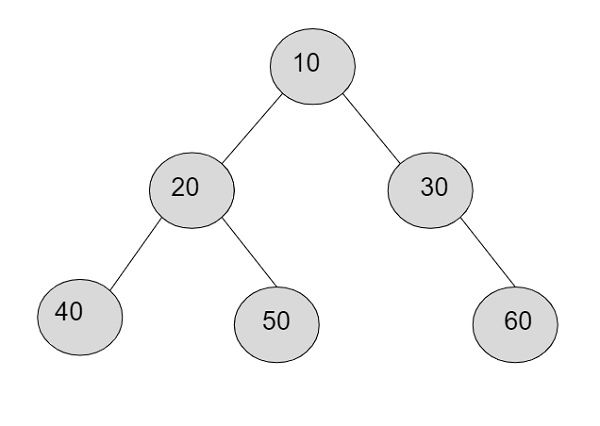# Product of nodes at k-th level in a tree represented as string in C++

Given with the tree of nodes with data in a string format and the task is to find the product of the nodes at k-th level in a binary tree. Every node of a tree contains three things i.e. data part, left pointer for left subtree and right pointer for right subtree.

Level of binary tree starts from number 0 and it can go till ‘n’ which can be any positive number. So, we are given with the level ‘k’ and program must calculate the product of the nodes at given ‘k’ level.

In the binary tree, if let’s say we are given with the value of k=2

So nodes at level 2 are − 40, 50, 60

Product= 40*50*60 = 1,20,000Input

(1(2(3()())(4()(5()())))(6(7()())(8()())))
K = 1

Output

product of nodes at level k = 12

Input

(0(5(6()())(4()(9()())))(7(1()())(3()())))"
k = 2

Output

product of nodes at level k = 72

## Algorithm

Start
Step 1→ Declare function to calculate nodes at k-th level
int product(string tree, int k)
Declare int level = -1
Declare int product = 1
Declare int size = tree.length()
Loop For int i = 0 and i < size and i++
IF tree[i] = '('
Set level++
End
Else IF tree[i] = ')'
Set level—
End
Else
IF level = k
Set product *= (tree[i] - '0')
End
End
End
return product
Step 2→ In main()
Declare string tree = "(1(2(3()())(4()(5()())))(6(7()())(8()())))"
Declare int k = 1
Call product(tree, k)
Stop

## Example

Live Demo

#include <bits/stdc++.h>
using namespace std;
//finding product at kth level
int product(string tree, int k){
int level = -1;
int product = 1;
int size = tree.length();
for (int i = 0; i < size; i++){
if (tree[i] == '(')
level++;
else if (tree[i] == ')')
level--;
else{
if (level == k)
product *= (tree[i] - '0');
}
}
return product;
}
int main(){
string tree = "(1(2(3()())(4()(5()())))(6(7()())(8()())))";
int k = 1;
cout <<"product of nodes at level k = "<<product(tree, k);
return 0;
}

## Output

If run the above code it will generate the following output −

product of nodes at level k = 12